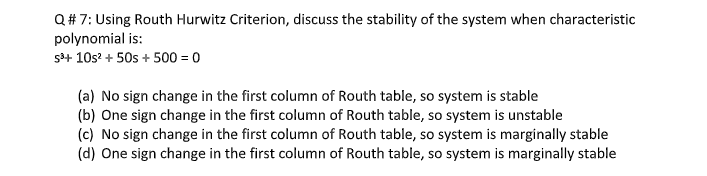# Question Q#7: Using Routh Hurwitz Criterion, discuss the stability of the system when characteristic polynomial is: S8+ 10s? + 50s + 500 = 0 (a) No sign change in the first column of Routh table, so system is stable (b) One sign change in the first column of Routh table, so system is unstable (c) No sign change in the first column of Routh table, so system is marginally stable (d) One sign change in the first column of Routh table, so system is marginally stable7QPLED The Asker · Electrical EngineeringTranscribed Image Text: Q#7: Using Routh Hurwitz Criterion, discuss the stability of the system when characteristic polynomial is: S8+ 10s? + 50s + 500 = 0 (a) No sign change in the first column of Routh table, so system is stable (b) One sign change in the first column of Routh table, so system is unstable (c) No sign change in the first column of Routh table, so system is marginally stable (d) One sign change in the first column of Routh table, so system is marginally stable
More
Transcribed Image Text: Q#7: Using Routh Hurwitz Criterion, discuss the stability of the system when characteristic polynomial is: S8+ 10s? + 50s + 500 = 0 (a) No sign change in the first column of Routh table, so system is stable (b) One sign change in the first column of Routh table, so system is unstable (c) No sign change in the first column of Routh table, so system is marginally stable (d) One sign change in the first column of Routh table, so system is marginally stable